# Theory of cost

30 de Sep de 2018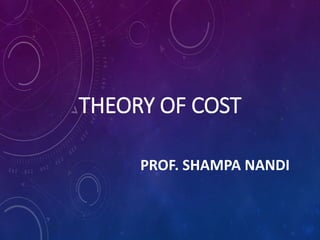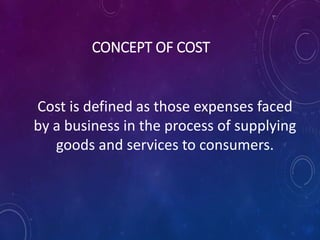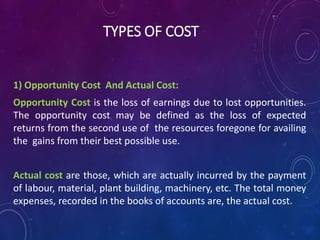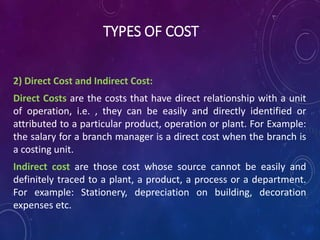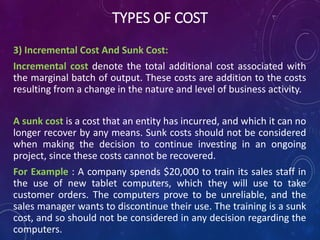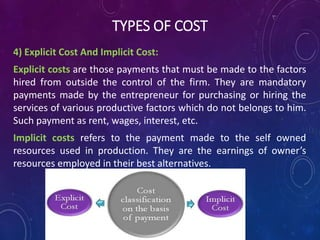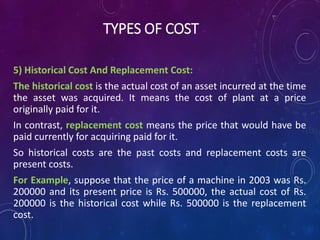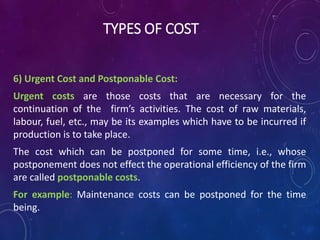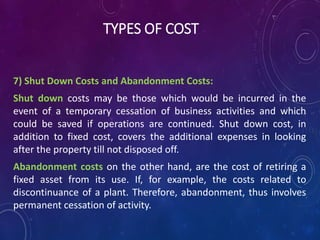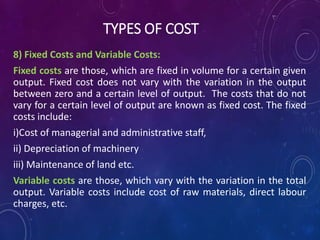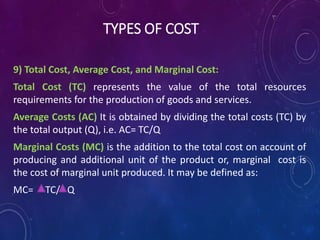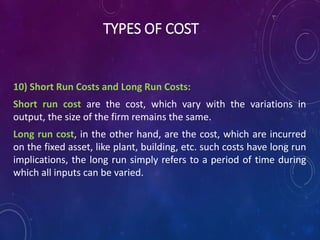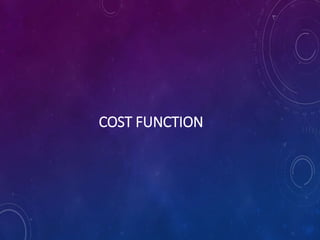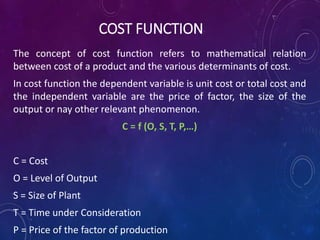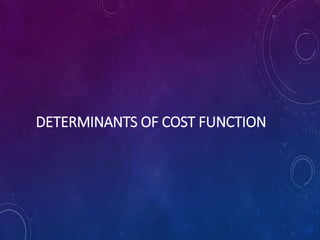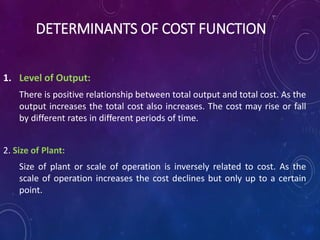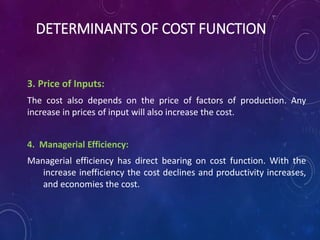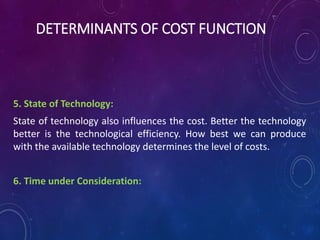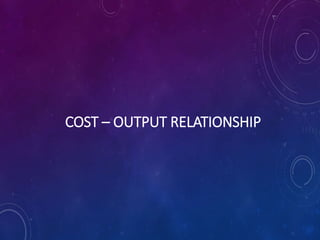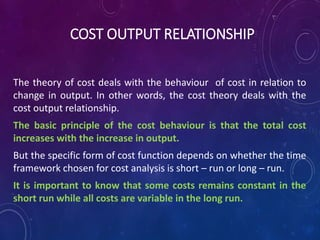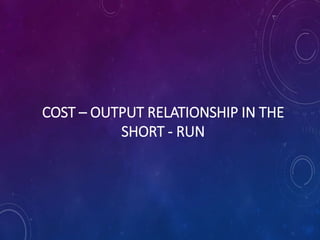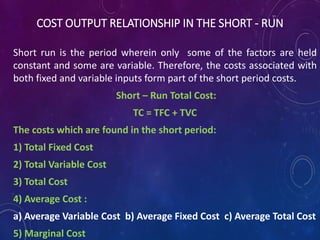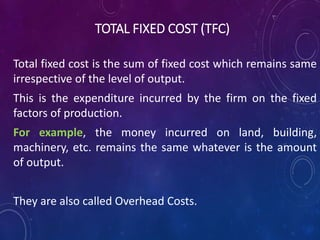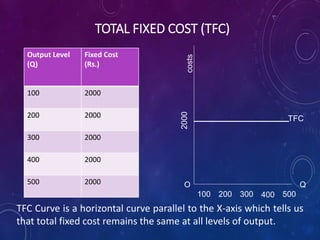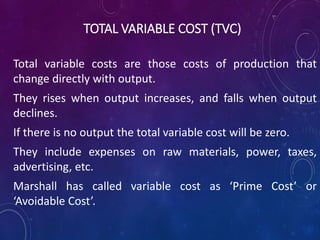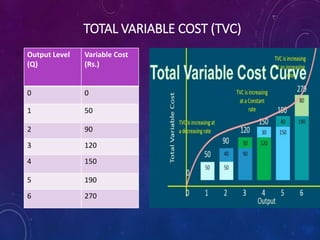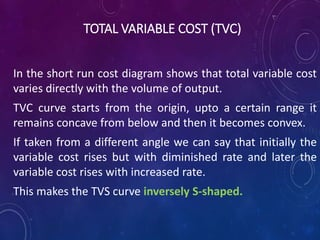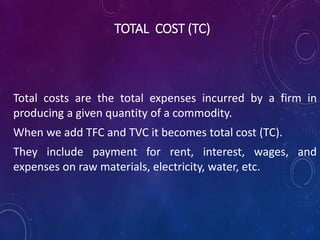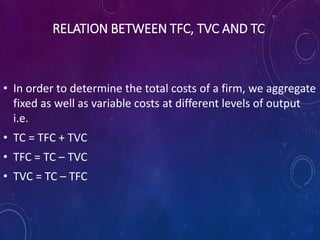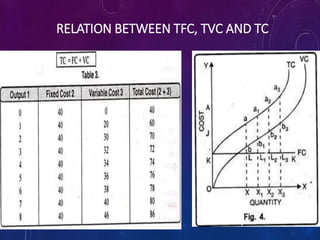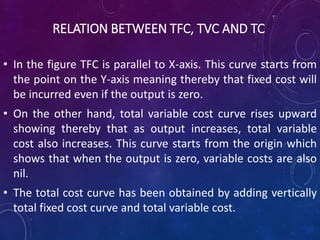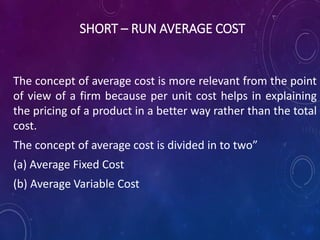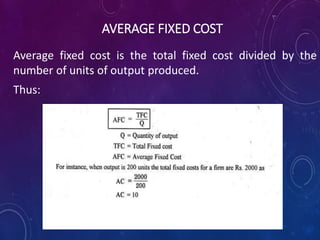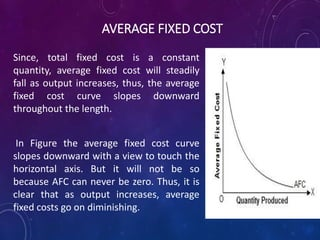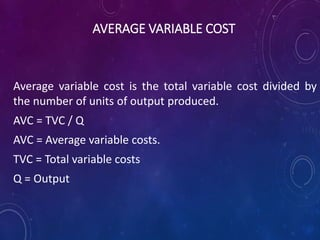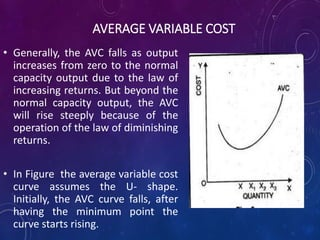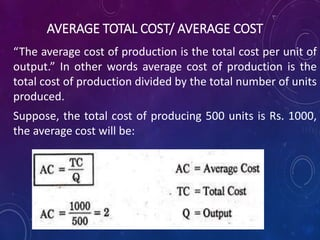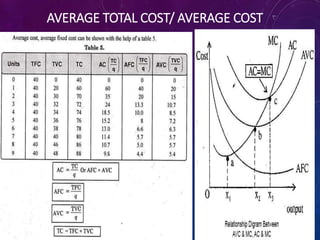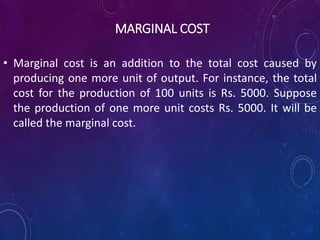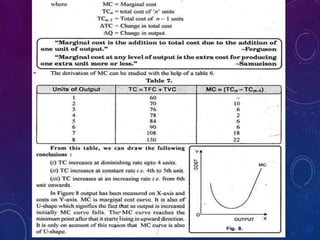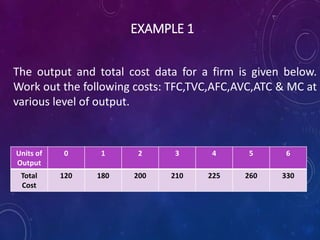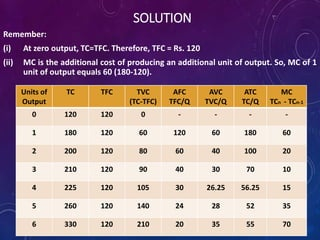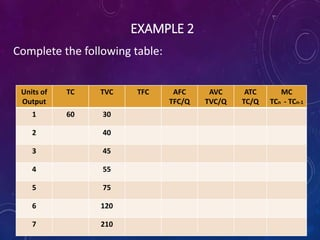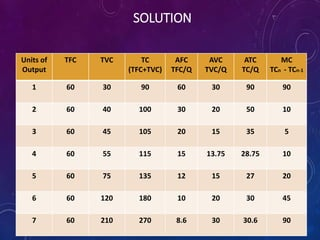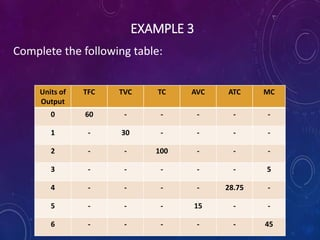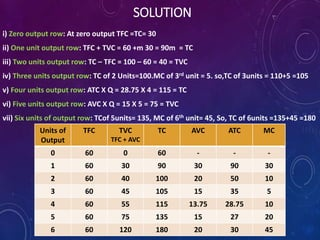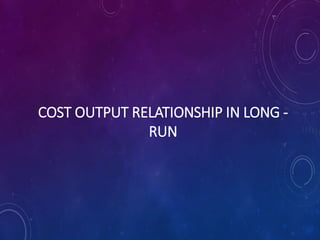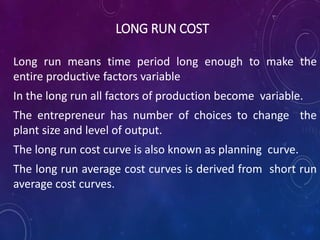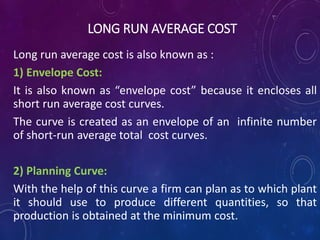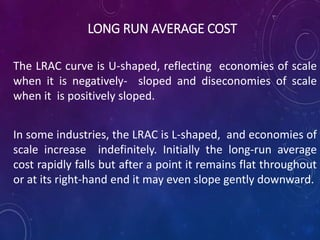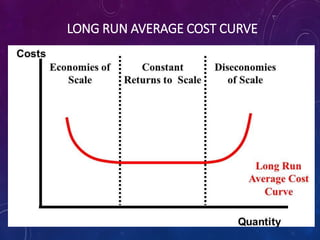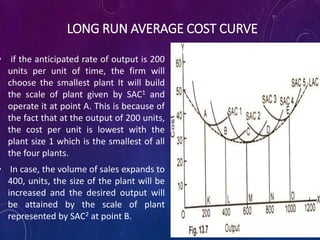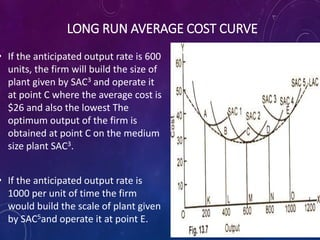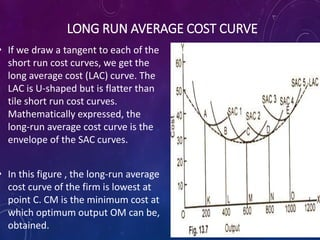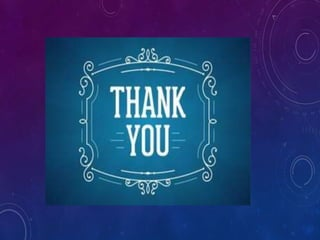1 de 56

### Theory of cost

• 1. THEORY OF COST PROF. SHAMPA NANDI
• 2. CONCEPT OF COST Cost is defined as those expenses faced by a business in the process of supplying goods and services to consumers.
• 3. TYPES OF COST 1) Opportunity Cost And Actual Cost: Opportunity Cost is the loss of earnings due to lost opportunities. The opportunity cost may be defined as the loss of expected returns from the second use of the resources foregone for availing the gains from their best possible use. Actual cost are those, which are actually incurred by the payment of labour, material, plant building, machinery, etc. The total money expenses, recorded in the books of accounts are, the actual cost.
• 4. TYPES OF COST 2) Direct Cost and Indirect Cost: Direct Costs are the costs that have direct relationship with a unit of operation, i.e. , they can be easily and directly identified or attributed to a particular product, operation or plant. For Example: the salary for a branch manager is a direct cost when the branch is a costing unit. Indirect cost are those cost whose source cannot be easily and definitely traced to a plant, a product, a process or a department. For example: Stationery, depreciation on building, decoration expenses etc.
• 5. TYPES OF COST 3) Incremental Cost And Sunk Cost: Incremental cost denote the total additional cost associated with the marginal batch of output. These costs are addition to the costs resulting from a change in the nature and level of business activity. A sunk cost is a cost that an entity has incurred, and which it can no longer recover by any means. Sunk costs should not be considered when making the decision to continue investing in an ongoing project, since these costs cannot be recovered. For Example : A company spends \$20,000 to train its sales staff in the use of new tablet computers, which they will use to take customer orders. The computers prove to be unreliable, and the sales manager wants to discontinue their use. The training is a sunk cost, and so should not be considered in any decision regarding the computers.
• 6. TYPES OF COST 4) Explicit Cost And Implicit Cost: Explicit costs are those payments that must be made to the factors hired from outside the control of the firm. They are mandatory payments made by the entrepreneur for purchasing or hiring the services of various productive factors which do not belongs to him. Such payment as rent, wages, interest, etc. Implicit costs refers to the payment made to the self owned resources used in production. They are the earnings of owner’s resources employed in their best alternatives.
• 7. TYPES OF COST 5) Historical Cost And Replacement Cost: The historical cost is the actual cost of an asset incurred at the time the asset was acquired. It means the cost of plant at a price originally paid for it. In contrast, replacement cost means the price that would have be paid currently for acquiring paid for it. So historical costs are the past costs and replacement costs are present costs. For Example, suppose that the price of a machine in 2003 was Rs. 200000 and its present price is Rs. 500000, the actual cost of Rs. 200000 is the historical cost while Rs. 500000 is the replacement cost.
• 8. TYPES OF COST 6) Urgent Cost and Postponable Cost: Urgent costs are those costs that are necessary for the continuation of the firm’s activities. The cost of raw materials, labour, fuel, etc., may be its examples which have to be incurred if production is to take place. The cost which can be postponed for some time, i.e., whose postponement does not effect the operational efficiency of the firm are called postponable costs. For example: Maintenance costs can be postponed for the time being.
• 9. TYPES OF COST 7) Shut Down Costs and Abandonment Costs: Shut down costs may be those which would be incurred in the event of a temporary cessation of business activities and which could be saved if operations are continued. Shut down cost, in addition to fixed cost, covers the additional expenses in looking after the property till not disposed off. Abandonment costs on the other hand, are the cost of retiring a fixed asset from its use. If, for example, the costs related to discontinuance of a plant. Therefore, abandonment, thus involves permanent cessation of activity.
• 10. TYPES OF COST 8) Fixed Costs and Variable Costs: Fixed costs are those, which are fixed in volume for a certain given output. Fixed cost does not vary with the variation in the output between zero and a certain level of output. The costs that do not vary for a certain level of output are known as fixed cost. The fixed costs include: i)Cost of managerial and administrative staff, ii) Depreciation of machinery iii) Maintenance of land etc. Variable costs are those, which vary with the variation in the total output. Variable costs include cost of raw materials, direct labour charges, etc.
• 11. TYPES OF COST 9) Total Cost, Average Cost, and Marginal Cost: Total Cost (TC) represents the value of the total resources requirements for the production of goods and services. Average Costs (AC) It is obtained by dividing the total costs (TC) by the total output (Q), i.e. AC= TC/Q Marginal Costs (MC) is the addition to the total cost on account of producing and additional unit of the product or, marginal cost is the cost of marginal unit produced. It may be defined as: MC= TC/ Q
• 12. TYPES OF COST 10) Short Run Costs and Long Run Costs: Short run cost are the cost, which vary with the variations in output, the size of the firm remains the same. Long run cost, in the other hand, are the cost, which are incurred on the fixed asset, like plant, building, etc. such costs have long run implications, the long run simply refers to a period of time during which all inputs can be varied.
• 14. COST FUNCTION The concept of cost function refers to mathematical relation between cost of a product and the various determinants of cost. In cost function the dependent variable is unit cost or total cost and the independent variable are the price of factor, the size of the output or nay other relevant phenomenon. C = f (O, S, T, P,…) C = Cost O = Level of Output S = Size of Plant T = Time under Consideration P = Price of the factor of production
• 16. DETERMINANTS OF COST FUNCTION 1. Level of Output: There is positive relationship between total output and total cost. As the output increases the total cost also increases. The cost may rise or fall by different rates in different periods of time. 2. Size of Plant: Size of plant or scale of operation is inversely related to cost. As the scale of operation increases the cost declines but only up to a certain point.
• 17. DETERMINANTS OF COST FUNCTION 3. Price of Inputs: The cost also depends on the price of factors of production. Any increase in prices of input will also increase the cost. 4. Managerial Efficiency: Managerial efficiency has direct bearing on cost function. With the increase inefficiency the cost declines and productivity increases, and economies the cost.
• 18. DETERMINANTS OF COST FUNCTION 5. State of Technology: State of technology also influences the cost. Better the technology better is the technological efficiency. How best we can produce with the available technology determines the level of costs. 6. Time under Consideration:
• 19. COST – OUTPUT RELATIONSHIP
• 20. COST OUTPUT RELATIONSHIP The theory of cost deals with the behaviour of cost in relation to change in output. In other words, the cost theory deals with the cost output relationship. The basic principle of the cost behaviour is that the total cost increases with the increase in output. But the specific form of cost function depends on whether the time framework chosen for cost analysis is short – run or long – run. It is important to know that some costs remains constant in the short run while all costs are variable in the long run.
• 21. COST – OUTPUT RELATIONSHIP IN THE SHORT - RUN
• 22. COST OUTPUT RELATIONSHIP IN THE SHORT - RUN Short run is the period wherein only some of the factors are held constant and some are variable. Therefore, the costs associated with both fixed and variable inputs form part of the short period costs. Short – Run Total Cost: TC = TFC + TVC The costs which are found in the short period: 1) Total Fixed Cost 2) Total Variable Cost 3) Total Cost 4) Average Cost : a) Average Variable Cost b) Average Fixed Cost c) Average Total Cost 5) Marginal Cost
• 23. TOTAL FIXED COST (TFC) Total fixed cost is the sum of fixed cost which remains same irrespective of the level of output. This is the expenditure incurred by the firm on the fixed factors of production. For example, the money incurred on land, building, machinery, etc. remains the same whatever is the amount of output. They are also called Overhead Costs.
• 24. TOTAL FIXED COST (TFC) Output Level (Q) Fixed Cost (Rs.) 100 2000 200 2000 300 2000 400 2000 500 2000 TFC Q costs 2000O 100 200 300 500400 TFC Curve is a horizontal curve parallel to the X-axis which tells us that total fixed cost remains the same at all levels of output.
• 25. TOTAL VARIABLE COST (TVC) Total variable costs are those costs of production that change directly with output. They rises when output increases, and falls when output declines. If there is no output the total variable cost will be zero. They include expenses on raw materials, power, taxes, advertising, etc. Marshall has called variable cost as ‘Prime Cost’ or ‘Avoidable Cost’.
• 26. TOTAL VARIABLE COST (TVC) Output Level (Q) Variable Cost (Rs.) 0 0 1 50 2 90 3 120 4 150 5 190 6 270
• 27. TOTAL VARIABLE COST (TVC) In the short run cost diagram shows that total variable cost varies directly with the volume of output. TVC curve starts from the origin, upto a certain range it remains concave from below and then it becomes convex. If taken from a different angle we can say that initially the variable cost rises but with diminished rate and later the variable cost rises with increased rate. This makes the TVS curve inversely S-shaped.
• 28. TOTAL COST (TC) Total costs are the total expenses incurred by a firm in producing a given quantity of a commodity. When we add TFC and TVC it becomes total cost (TC). They include payment for rent, interest, wages, and expenses on raw materials, electricity, water, etc.
• 29. RELATION BETWEEN TFC, TVC AND TC • In order to determine the total costs of a firm, we aggregate fixed as well as variable costs at different levels of output i.e. • TC = TFC + TVC • TFC = TC – TVC • TVC = TC – TFC
• 30. RELATION BETWEEN TFC, TVC AND TC
• 31. RELATION BETWEEN TFC, TVC AND TC • In the figure TFC is parallel to X-axis. This curve starts from the point on the Y-axis meaning thereby that fixed cost will be incurred even if the output is zero. • On the other hand, total variable cost curve rises upward showing thereby that as output increases, total variable cost also increases. This curve starts from the origin which shows that when the output is zero, variable costs are also nil. • The total cost curve has been obtained by adding vertically total fixed cost curve and total variable cost.
• 32. SHORT – RUN AVERAGE COST The concept of average cost is more relevant from the point of view of a firm because per unit cost helps in explaining the pricing of a product in a better way rather than the total cost. The concept of average cost is divided in to two” (a) Average Fixed Cost (b) Average Variable Cost
• 33. AVERAGE FIXED COST Average fixed cost is the total fixed cost divided by the number of units of output produced. Thus:
• 34. AVERAGE FIXED COST Since, total fixed cost is a constant quantity, average fixed cost will steadily fall as output increases, thus, the average fixed cost curve slopes downward throughout the length. In Figure the average fixed cost curve slopes downward with a view to touch the horizontal axis. But it will not be so because AFC can never be zero. Thus, it is clear that as output increases, average fixed costs go on diminishing.
• 35. AVERAGE VARIABLE COST Average variable cost is the total variable cost divided by the number of units of output produced. AVC = TVC / Q AVC = Average variable costs. TVC = Total variable costs Q = Output
• 36. AVERAGE VARIABLE COST • Generally, the AVC falls as output increases from zero to the normal capacity output due to the law of increasing returns. But beyond the normal capacity output, the AVC will rise steeply because of the operation of the law of diminishing returns. • In Figure the average variable cost curve assumes the U- shape. Initially, the AVC curve falls, after having the minimum point the curve starts rising.
• 37. AVERAGE TOTAL COST/ AVERAGE COST “The average cost of production is the total cost per unit of output.” In other words average cost of production is the total cost of production divided by the total number of units produced. Suppose, the total cost of producing 500 units is Rs. 1000, the average cost will be:
• 38. AVERAGE TOTAL COST/ AVERAGE COST
• 39. MARGINAL COST • Marginal cost is an addition to the total cost caused by producing one more unit of output. For instance, the total cost for the production of 100 units is Rs. 5000. Suppose the production of one more unit costs Rs. 5000. It will be called the marginal cost.
• 41. FEW PROBLEMS TO PRACTICE
• 42. EXAMPLE 1 The output and total cost data for a firm is given below. Work out the following costs: TFC,TVC,AFC,AVC,ATC & MC at various level of output. Units of Output 0 1 2 3 4 5 6 Total Cost 120 180 200 210 225 260 330
• 43. SOLUTION Remember: (i) At zero output, TC=TFC. Therefore, TFC = Rs. 120 (ii) MC is the additional cost of producing an additional unit of output. So, MC of 1 unit of output equals 60 (180-120). Units of Output TC TFC TVC (TC-TFC) AFC TFC/Q AVC TVC/Q ATC TC/Q MC TCn - TCn-1 0 120 120 0 - - - - 1 180 120 60 120 60 180 60 2 200 120 80 60 40 100 20 3 210 120 90 40 30 70 10 4 225 120 105 30 26.25 56.25 15 5 260 120 140 24 28 52 35 6 330 120 210 20 35 55 70
• 44. EXAMPLE 2 Complete the following table: Units of Output TC TVC TFC AFC TFC/Q AVC TVC/Q ATC TC/Q MC TCn - TCn-1 1 60 30 2 40 3 45 4 55 5 75 6 120 7 210
• 45. SOLUTION Units of Output TFC TVC TC (TFC+TVC) AFC TFC/Q AVC TVC/Q ATC TC/Q MC TCn - TCn-1 1 60 30 90 60 30 90 90 2 60 40 100 30 20 50 10 3 60 45 105 20 15 35 5 4 60 55 115 15 13.75 28.75 10 5 60 75 135 12 15 27 20 6 60 120 180 10 20 30 45 7 60 210 270 8.6 30 30.6 90
• 46. EXAMPLE 3 Complete the following table: Units of Output TFC TVC TC AVC ATC MC 0 60 - - - - - 1 - 30 - - - - 2 - - 100 - - - 3 - - - - - 5 4 - - - - 28.75 - 5 - - - 15 - - 6 - - - - - 45
• 47. SOLUTION i) Zero output row: At zero output TFC =TC= 30 ii) One unit output row: TFC + TVC = 60 +m 30 = 90m = TC iii) Two units output row: TC – TFC = 100 – 60 = 40 = TVC iv) Three units output row: TC of 2 Units=100.MC of 3rd unit = 5. so,TC of 3units = 110+5 =105 v) Four units output row: ATC X Q = 28.75 X 4 = 115 = TC vi) Five units output row: AVC X Q = 15 X 5 = 75 = TVC vii) Six units of output row: TCof 5units= 135, MC of 6th unit= 45, So, TC of 6units =135+45 =180 Units of Output TFC TVC TFC + AVC TC AVC ATC MC 0 60 0 60 - - - 1 60 30 90 30 90 30 2 60 40 100 20 50 10 3 60 45 105 15 35 5 4 60 55 115 13.75 28.75 10 5 60 75 135 15 27 20 6 60 120 180 20 30 45
• 48. COST OUTPUT RELATIONSHIP IN LONG - RUN
• 49. LONG RUN COST Long run means time period long enough to make the entire productive factors variable In the long run all factors of production become variable. The entrepreneur has number of choices to change the plant size and level of output. The long run cost curve is also known as planning curve. The long run average cost curves is derived from short run average cost curves.
• 50. LONG RUN AVERAGE COST Long run average cost is also known as : 1) Envelope Cost: It is also known as “envelope cost” because it encloses all short run average cost curves. The curve is created as an envelope of an infinite number of short-run average total cost curves. 2) Planning Curve: With the help of this curve a firm can plan as to which plant it should use to produce different quantities, so that production is obtained at the minimum cost.
• 51. LONG RUN AVERAGE COST The LRAC curve is U-shaped, reflecting economies of scale when it is negatively- sloped and diseconomies of scale when it is positively sloped. In some industries, the LRAC is L-shaped, and economies of scale increase indefinitely. Initially the long-run average cost rapidly falls but after a point it remains flat throughout or at its right-hand end it may even slope gently downward.
• 52. LONG RUN AVERAGE COST CURVE
• 53. LONG RUN AVERAGE COST CURVE • if the anticipated rate of output is 200 units per unit of time, the firm will choose the smallest plant It will build the scale of plant given by SAC1 and operate it at point A. This is because of the fact that at the output of 200 units, the cost per unit is lowest with the plant size 1 which is the smallest of all the four plants. • In case, the volume of sales expands to 400, units, the size of the plant will be increased and the desired output will be attained by the scale of plant represented by SAC2 at point B.
• 54. LONG RUN AVERAGE COST CURVE • If the anticipated output rate is 600 units, the firm will build the size of plant given by SAC3 and operate it at point C where the average cost is \$26 and also the lowest The optimum output of the firm is obtained at point C on the medium size plant SAC3. • If the anticipated output rate is 1000 per unit of time the firm would build the scale of plant given by SAC5and operate it at point E.
• 55. LONG RUN AVERAGE COST CURVE • If we draw a tangent to each of the short run cost curves, we get the long average cost (LAC) curve. The LAC is U-shaped but is flatter than tile short run cost curves. Mathematically expressed, the long-run average cost curve is the envelope of the SAC curves. • In this figure , the long-run average cost curve of the firm is lowest at point C. CM is the minimum cost at which optimum output OM can be, obtained.